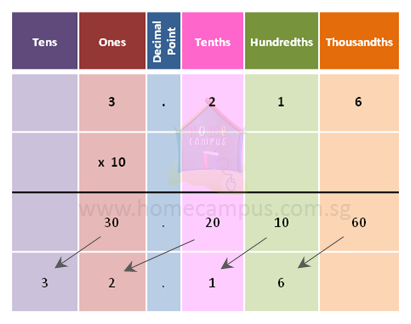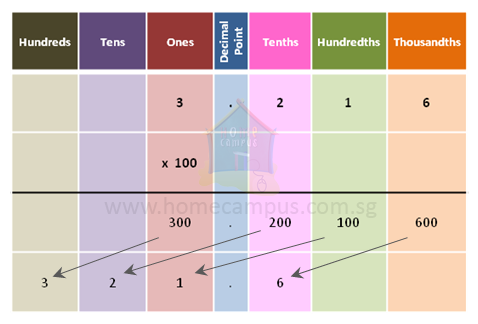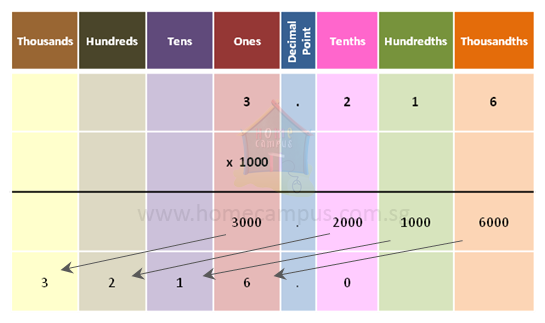## How to Multiply Decimal Numbers by 10s, 100s and 1000s (Powers of 10)?

Practice Unlimited Questions

#### 1.  Find the product of 3.216 and 10.3.216  ×  10  =  32.16
When you multiply a decimal number by 10, its decimal point moves 1 place to the right.

Quick trick: Since there is 1 zero in 10, move the decimal point 1 place to the right.

#### 2.  Find the product of 3.216 and 100.3.216  ×  100  =  321.6
When you multiply a decimal number by 100, its decimal point moves 2 places to the right.

Quick trick: Since there are 2 zeroes in 100, move the decimal point 2 places to the right.

#### 3.  Find the product of 3.216 and 1000.3.216  ×  1000  =  3216.0  =  3216
When you multiply a decimal number by 1000, its decimal point moves 3 places to the right.

Quick trick: Since there are 3 zeroes in 1000, move the decimal point 3 places to the right.

#### 4.  What is 3.216  ×  200?

3.216  ×  200
=  3.216  ×  2  ×  100
=  6.432  ×  100
=  643.2
200  =  2  ×  100

3.216  ×  2  =  6.432